### Home > CALC > Chapter 11 > Lesson 11.1.3 > Problem11-34

11-34.
1.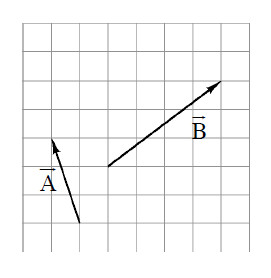For each vector drawn at right: Homework Help ✎

1. Rewrite the vector in both component and i, j form.

2. Find each vector's standard angle.

3. Find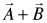and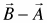.

4. Determine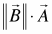.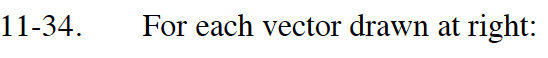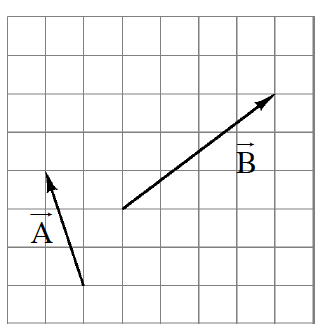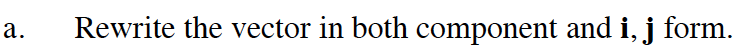A = –1i + 3j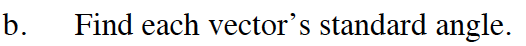Solve tan(θ) = 3/1. This will solve for the angle in the slope triangle for A.
Recall that the standard angle is measured from the positive x-axis.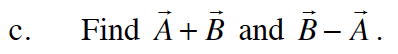A + B = 〈 –1, 3〉 + 〈 4, 3〉 = ?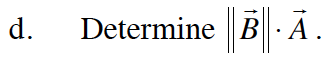The double bars mean "the magnitude of". To calculate the magnitude of vector B, use the Pythagorean Theorem.